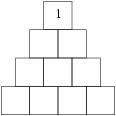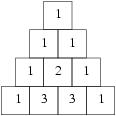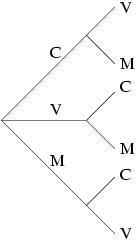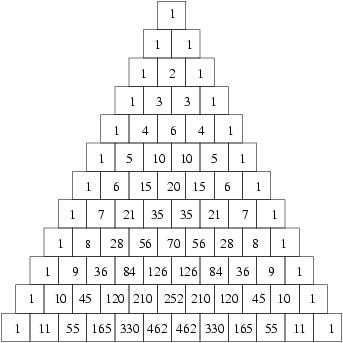### Pascal's Triangle

Blaise Pascal was a French mathematician who lived in the 17th century. He made several important advances in geometry, but perhaps his most famous creation was a triangle that isn't really a triangle. Called 'Pascal's Triangle,' it has many angles (so to speak), which we can take a look at, but first let's see what it is. The way to make the triangle is very simple. First, draw boxes like this, and put a 1 at the top:Now, fill in each box by adding up the two boxes directly above it and putting the result in the box. If there's only one box above it, just copy that number into the box. In the end, your diagram should look like this:The 1's down the side are there because above each of those boxes, there is only one box, with just a 1 in it. The 3 is there because above it is a 1 and a 2, which when added together make 3.

The first thing that was noticed about Pascal's Triangle is how useful it is in a part of maths called combinatorics. Like lots of long words, giving an example is the best way to explain what it means. Suppose you want to buy an ice cream. The shop has a special, so you decide to get two ice creams. There are 3 flavours available: chocolate, vanilla, and mango. You don't want to get two of the same flavour. How many possibilities are there for which ice creams you get?

The first thing to do in problems like this is usually to make a chart, so let's do that. If C stands for chocolate, V for vanilla, and M for mango, you could get 6 possibilities:

CV CM VC VM MC MV

However, something's not quite right. After all, does it really matter if your first ice cream is chocolate and your second one vanilla, or first vanilla and then chocolate? Not unless you're an ice cream fanatic! So let's say that CV (chocolate then vanilla) is the same as VC (vanilla then chocolate). In that case, those 6 choices are actually only 3 : CV, CM, and VM. Notice that exactly half the possibilities disappeared, since they were just the reverse of the first half : MC is the opposite of CM.

So that's combinatorics : figuring out the number of ways that something can happen. It's called that because those 3 different possibilities are called combinations : different ways to combine things. Those 6 possibilities are called permutations : that's what you call them when the order matters, like if chocolate-mango isn't the same thing at all as mango-chocolate. Why, you may be asking, do we need a special word for all of this? And why do we need that weird triangle up there? Well, let's try another example.

Let's say you're the manager of a cricket team, and you need to choose an opening pair. Now, all of the players are very selfish, and they all want to open. As well, they all have their preferences in opening partners. So you tell them that, to avoid favouritism, you will make every possible opening pair the opening pair in one innings. The question you have to ask now is, how many possible opening pairs are there? With 11 players, making a list isn't so simple any more (try if you don't believe me!). Maybe there's more to this combinatorics stuff than meets the eye.

The first thing mathematicians do when they have a tough problem is to try and reduce it to a simpler problem, one they already know how to solve. We solved that ice cream problem, but we did it using a list, which doesn't look like it'll do us that much good in the long run. So let's take another taste of the ice cream.

The first thing we need to do is choose our first ice cream flavour. There are 3 choices there. Next we have to choose our second flavour. Since we already chose one, that leaves 2 choices. Since there are 3 choices, then 2 choices, we actually have 6 ways to go (check out that list above if you don't believe me). Why? Well, think of our choices as roads we can take. We start at a point and have three directions to choose. Then, each of those directions splits into two more. So we have 3 2's, or in other words, 3X2. Here's a picture:But remember, we really don't care about the difference between chocolate-vanilla and vanilla-chocolate. That means that every possibility we have actually appears twice : frontwards and backwards. So we have to divide by 2. 3 X 2/2 = 3, so that's our answer.

This teaches us a valuable lesson in how to calculate combinations. We're going to need to multiply to figure out the number of ways we can do something, and then divide if we get any duplicate ways : two different ways that are really the same. Let's look at one more example.

Suppose we have 5 flavours now : our old chocolate, vanilla, and mango, and new strawberry and pista. And let's say that this time we want 3 ice creams (getting a little greedy). How many ways can we choose our flavours?

Well, let's think about this in our new way. For our first ice cream, we can choose any one of the 5. For our second ice cream, we can choose any one of the 4 remaining flavours, so if we chose vanilla first, now we can choose any one of chocolate, mango, strawberry, or pista. For our third and final flavour, we can choose any one of the remaining 3. So first we choose between 5 flavours, then each of those five paths leads to 4 new choices, and then each of those 4 leads to 3 new ones. That gives us 5 X 4 X 3= 60. (Try drawing a tree if you're not sure how this works.) But wait, we're counting chocolate-vanilla-mango and chocolate-mango-vanilla as different, when everybody knows they're the same. So how many different ways are there to put 3 flavours in different order? It turns out there are 6 (CVM, CMV, VCM, VMC, MCV, MVC are the six for those flavours), which means we have to divide by 6, since otherwise we'll be counting each possibility 6 times. Dividing 60 by 6 gives us 10, so there are 10 different ways to get 3 different ice cream flavours from 5 choices.

Now let's go back to cricket. For your first batsman, you have 11 choices. For the second, there are 10 men remaining, so you have 10 choices. 11 X 10=110. Now, assuming the batsmen don't care which of them takes the first over, you need to divide by two, since Ganguly-Tendulkar is the same as Tendulkar-Ganguly. 110/2=55, which means that your team will have to play 55 innings to try out every opening pair. Think your managerial career will last that long?

OK, you say, all this is well and good. Now I know how to get sacked as a cricket manager. But what on earth does that triangle up there have to do with any of this? Well, if you add a few rows to the triangle, you'll notice a curious fact : the answers we've come up with are in fact right there in the triangle.Look at the 5th row down, counting the top row (with just a 1 in it) as the 0th row. The 5th row is '1 5 10 10 5 1.' Now count over on this row, with the left-most number being the 0th number. The 0th is 1, the 1st is 5, 2nd is 10, and 3rd is 10. (Yes, the 0?s make things confusing.) Remember what our answer was for 3 choices from 5 ice cream flavours? It was 10, same as here (for the 5th row, 3rd column). Now, go to the 11th row (again, the top row is 0th), and count over 2 from the left (again, left-most is 0th) to get the number of opening pairs you can have. Remember, all you need to do for the Pascal's triangle is to add 2 numbers together, not multiply and divide lots of numbers like we were doing before. Easy, isn't it ?

Next time, we'll see how Pascal's Triangle works, and also that when you look at it from a long way away, all the numbers become even!

-Janak Ramakrishnan, Chennai